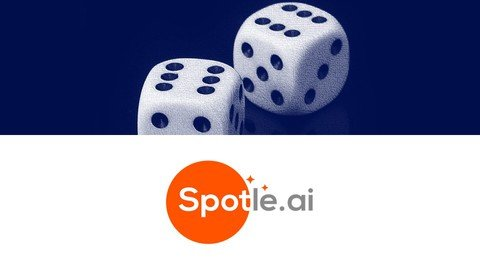## Probability And Distribution With Solved Problems By SpotleProbability And Distribution With Solved Problems By Spotle
Published 8/2022
MP4 | Video: h264, 1280x720 | Audio: AAC, 44.1 KHz
Language: English | Size: 553.34 MB | Duration: 1h 30m

Learn probability and distribution explained in detail with solved problems and exercises
What you'll learn
Learn probability, conditional probability, Bayes' theorem, and basic distribution explained through detailed videos
Learn random variables - continuous and discrete variables
Learn how to solve probability problems
Learn through exercises and solved problems
Requirements
In this Spotle compact course you will start from scratch
Description
One of the most talked about career options today is a career in Data Science. In this Spotle compact course we focus on building the foundation solid. To become a data scientist or a machine learning expert, it is essential to learn the concepts of probability. You need to be ready to apply the concepts in real-life problem solving. The concepts will be quite frequently applied in almost all areas of Data Science and Machine Learning.Probability, random variables, and probability distributions form the foundation of building complex algorithms.This course is also highly recommended for the students of standard 12 to graduation level who are dong major in Statistics, mathematics or in any tech based course such as biotech. Each and every topic in this Spotle compact course has been explained with multiple examples.At the end 30 problems have been explained in detail and solved. 20 exercises have been added to help you check that you have understood the topics very well. Practice as much as you can.In all Spotle compact courses we have created the videos so that you will need minimum time to learn, so that you can give more time to applying them in real-life.
Overview
Section 1: Introduction
Lecture 1 The Probability Concepts
Lecture 2 The Conditional Probability Concepts
Section 2: Conditional Probability
Lecture 3 Conditional Probability
Lecture 4 Bayes Theorem And Conditional Probability
Section 3: Random Variables
Lecture 5 What Is A Random Variable
Lecture 6 Random Variables - An Exercise
Lecture 7 Types Of Random Variables
Section 4: Probability Distribution
Lecture 8 Probability Mass And Density Function
Lecture 9 Uniform Distribution And Cumulative Distribution Function
Section 5: Expectations And Variance
Lecture 10 Calculating Expectations
Lecture 11 Calculating Variance
Section 6: Probability Distribution With Python
Lecture 12 Probability Distribution With Python
Section 7: Data Science/ Machine Learning Applications
Lecture 13 Naïve Bayes Classifier
Section 8: Solved Problems
Lecture 14 Probability Quiz - 10 Solved Problems With Detailed Explanation
Lecture 15 The Tricky Probability Test - 10 Solved Problems
Lecture 16 Random Variables And Distribution - 10 Solved Problems
Section 9: Exercise - 20 Problems On Probability And Permutation
Lecture 17 Exercise - 20 Problems On Probability And Permutation
This is an ideal course for the people who want to build a rewarding career in Data Science.,This course is highly recommended for the students of standard 12 to graduation level who are dong major in science.,This course helps learn probability concepts needed pretty much for any tech based course.
Screenshots

#